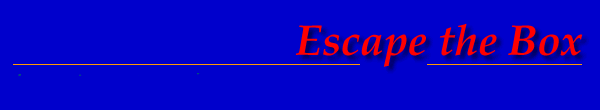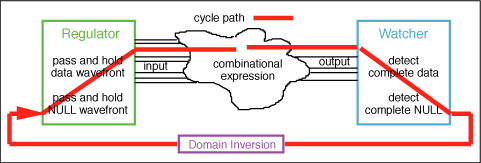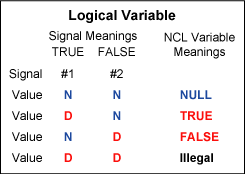Theseus Research : Technical Papers : Considerations of Completeness Page 6 of 11

The Time Relationship

The feedback path R must stabilize strictly before the next wavefront is presented to the gate. The presentation of the next wavefront will not occur until the current wavefront has flowed through the combinational expression and through the watcher, the acknowledge signal has flowed to the regulator and the regulator has allowed the next wavefront to flow into the combinational expression. This will be referred to as the NCL cycle path shown in Figure 9. The cycle path propagation time for a particular expression is the cycle period of the expression. If the feedback signal path of a gate is slower than the cycle period of the expression, there is a critical hazard and the gate can behave incorrectly so this temporal relationship must be considered in the complete expression of behavior. However, this feedback path can be isolated inside the gate and its time relationship can be easily rendered insignificant in relation to the cycle period of the expression.

Figure 9. The NCL cycle path.The Compromise to Logical Completenesses

The completeness criterion and completeness of participation are still intact but single-form completeness of expression has been compromised. The behavior of the expression is no longer characterized completely in terms of logical relationships. An expression of time must also be included to completely express the behavior of a combinational process. However, it is clear where in the expression of single form completeness has been compromised, it is clear that this compromise can be benignly isolated inside a gate and it is clear to what extent logical completeness can still be considered with advantage. Assuming effective isolation inside each gate, the input and output boundaries of the gates still form logically determined completeness boundaries that compose to logically determined combinational expressions just like 4NCL.

Relationship of 3NCL to Boolean Logic

3NCL still differentiates two data values and can be mapped gate for gate to Boolean logic expressions.

Can a 2 value logic be logically complete?

If the expressivity of the logic is reduced to only two values, it must be decided what those two values will differentiate. 4NCL and 3NCL differentiate data states from non-data states and also differentiate two data states. If only two logic values are available only one of these differentiations can be expressed.

If it is decided to differentiate two data values with the logical values such as TRUE and FALSE the resulting logic is Boolean logic. A Boolean logic expression constantly expresses data and cannot differentiate data from non-data. For a Boolean logic combinational expression the differentiation of successive data sets and the beginning and ending of a data set resolution must be expressed non-logically with some convention of time relationship such as a clock or delay element.

If it is decided to differentiate data from non-data, the NULL convention can be continued with the logical values DATA and NULL and the hysteresis behavior can be retained. The resulting logic is 2 value NULL Convention Logic (2NCL). In this case the differentiation of data values is no longer expressed logically and must be expressed non-logically with a multiple signal path data representation convention. Figure 10 shows the correspondences between all four logics.

Figure 10. Correspondence of the logics.

 4NCL 3NCL 2NCL Boolean Logic TRUE TRUE TRUE FALSE FALSE DATA FALSE INTERMEDIATE Hysteresis Behavior Hysteresis Behavior NULL NULL NULL

The Data Representation Convention

Since, in 2NCL, there is only one DATA value, each signal path in a 2NCL expression represents one specific meaning and the path either asserts its meaning (DATA) or does not assert its meaning (NULL). To represent a variable that can express multiple mutually exclusive values multiple signal paths must be used. To form a binary variable that mutually exclusively expresses TRUE and FALSE, for instance, there must be two signal paths, one signal path meaning TRUE and one signal path meaning FALSE. For any data wavefront only one of the two paths may express its DATA value. It is illegal for both DATA values to be simultaneously expressed. The two signal paths then form a single dual-rail variable that can express two mutually exclusive DATA values or NULL as shown in Figure 11.

Figure 11. 2 value NCL variable formed from two signal paths.In general, an N value NCL variable can be represented with N signal paths only one of which will assert a DATA value in a given data wavefront [reference 7]. Figure 12 shows several examples of NCL multi-value variables.

Figure 12. Multi path NCL variables.Each signal path is a value of a variable. This is in contrast to the familiar situation of each signal path constituting a whole variable. Variables of any size can be built from signal paths and arbitrary mutually exclusive meanings can be assigned to the paths. This property is useful for implementing higher radix and even mixed radix numeric functions and for control expressions. For instance, the familiar one-hot control code, derived typically from a decode, becomes a single large NCL variable. In this sense, 2NCL is a general multi-value logic.

For a data wavefront, each variable asserts exactly one DATA value. Completeness of the data wavefront is exactly one DATA value per variable. For a NULL wavefront all asserted DATA values return to NULL and completeness for a NULL wavefront is all NULL values across all variables.